NumPy is a Python library optimized for numerical computing. It bears close semblance with MATLAB and is equally as powerful when used in conjunction with other packages such as SciPy for various scientific functions, Matplotlib for visualization and Pandas for data analysis. NumPy is short for numerical python.

NumPy core strength lies in its ability to create and manipulate $n$-dimensional arrays. This is particularly critical for building Machine learning and Deep learning models. Data is often represented in a matrix-like grid of rows and columns, where each row represents an observation and each column a variable or feature. Hence, NumPy’s 2-Dimensional arrays is a natural fit for storing and manipulating datasets.

This tutorial will cover the basics of NumPy to get you very comfortable working with the package and also get you to appreciate the thinking behind how NumPy works. This understanding forms a foundation from which one can extend and seek solutions from the NumPy reference documentation when a specific functionality is needed.

To begin using NumPy, we’ll start by importing the NumPy module:

import numpy as np


### NumPy 1-D Array

Let’s create a simple 1-D NumPy array:

> my_array = np.array([2,4,6,8,10])
> my_array
'Output': array([ 2,  4,  6,  8, 10])
# the data-type of a NumPy array is the ndarray
> type(my_array)
'Output': numpy.ndarray
# a NumPy 1-D array can also be seen a vector with 1 dimension
> my_array.ndim
'Output': 1
# check the shape to get the number of rows and columns in the array \
> my_array.shape
'Output': (5,)


We can also create an array from a Python list

> my_list = [9, 5, 2, 7]
> type(my_list)
'Output': list
# convert a list to a numpy array
> list_to_array = np.array(my_list) # or np.asarray(my_list)
> type(list_to_array)
'Output': numpy.ndarray


Let’s explore other useful methods often employed for creating arrays

# create an array from a range of numbers
> np.arange(10)
'Output': [0 1 2 3 4 5 6 7 8 9]
# create an array from start to end (exclusive) via a step size - (start, stop, step)
> np.arange(2, 10, 2)
'Output': [2 4 6 8]
# create a range of points between two numbers
> np.linspace(2, 10, 5)
'Output': array([  2.,   4.,   6.,   8.,  10.])
# create an array of ones
> np.ones(5)
'Output': array([ 1.,  1.,  1.,  1.,  1.])
# create an array of zeros
> np.zeros(5)
'Output': array([ 0.,  0.,  0.,  0.,  0.])


### NumPy Datatypes

NumPy boasts a broad range of numerical datatypes in comparison with vanilla Python. This extended datatype support is useful for dealing with different kinds of signed and unsigned integer and floating-point numbers and well as booleans and complex numbers for scientific computation. NumPy datatypes include the bool_, int(8,16,32,64), uint(8,16,32,64), float(16,32,64), complex(64,128) as well as the int_, float_ and complex_ to mention just a few.

The datatypes with a _ appended are base Python datatypes converted to NumPy datatypes. The parameter dtype is used to assign a datatype to a NumPy function. The default NumPy type is float_. Also, Numpy infers contiguous arrays of the same type.

Let’s explore a bit with NumPy datatypes:

# ints
> my_ints = np.array([3, 7, 9, 11])
> my_ints.dtype
'Output': dtype('int64')

# floats
> my_floats = np.array([3., 7., 9., 11.])
> my_floats.dtype
'Output': dtype('float64')

# non-contiguous types - default: float
> my_array = np.array([3., 7., 9, 11])
> my_array.dtype
'Output': dtype('float64')

# manually assigning datatypes
> my_array = np.array([3, 7, 9, 11], dtype="float64")
> my_array.dtype
'Output': dtype('float64')


### Indexing + Fancy Indexing (1-D)

We can index a single element of a NumPy 1-D array similar to how we index a Python list.

# create a random numpy 1-D array
> my_array = np.random.rand(10)
> my_array
'Output': array([ 0.7736445 ,  0.28671796,  0.61980802,  0.42110553,  0.86091567,
0.93953255,  0.300224  ,  0.56579416,  0.58890282,  0.97219289])
# index the first element
> my_array
'Output': 0.77364449999999996
# index the last element
> my_array[-1]
'Output': 0.97219288999999998


Fancy Indexing in NumPy is an advanced mechanism for indexing array elements based on integers or boolean. This technique is also called masking.

Let’s index all the even integers in the array using a boolean mask.

# create 10 random integers between 1 and 20
> my_array = np.random.randint(1, 20, 10)
> my_array
'Output': array([14,  9,  3, 19, 16,  1, 16,  5, 13,  3])
# index all even integers in the array using a boolean mask
> my_array[my_array % 2 == 0]
'Output': array([14, 16, 16])


Observe that the code my_array % 2 == 0 output’s an array of booleans

> my_array % 2 == 0
'Output': array([ True, False, False, False,  True, False,  True, False, False, False], dtype=bool)


Let’s select all elements with even indices in the array.

# create 10 random integers between 1 and 20
> my_array = np.array([14,  9,  3, 19, 16,  1, 16,  5, 13,  3])
> my_array
'Output': array([14,  9,  3, 19, 16,  1, 16,  5, 13,  3])
> my_array[np.arange(1,10,2)]
'Output': array([ 9, 19,  1,  5,  3])


Remember that array indices are indexed from 0. So the second element, 9 is in the first index 1.

> np.arange(1,10,2)
'Output': array([1, 3, 5, 7, 9])


### Slicing a 1-D Array

Slicing a NumPy array is also similar to slicing a Python list.

> my_array = np.array([14,  9,  3, 19, 16,  1, 16,  5, 13,  3])
> my_array
'Output': array([14,  9,  3, 19, 16,  1, 16,  5, 13,  3])
# slice the first 2 elements
> my_array[:2]
'Output': array([14,  9])
# slice the last 3 elements
> my_array[-3:]
'Output': array([ 5, 13,  3])


### Basic Math Operations on Arrays: Universal Functions

The core power of NumPy is in its highly optimized vectorized functions for various mathematical, arithmetic and string operations. In NumPy these functions are called universal functions. We’ll explore a couple of basic arithmetic with NumPy 1-D arrays.

# create an array of even numbers between 2 and 10
> my_array = np.arange(2,11,2)
'Output': array([ 2,  4,  6,  8, 10])
# sum of array elements
> np.sum(my_array) # or my_array.sum()
'Output': 30
# square root
> np.sqrt(my_array)
'Output': array([ 1.41421356,  2.        ,  2.44948974,  2.82842712,  3.16227766])
# log
> np.log(my_array)
'Output': array([ 0.69314718,  1.38629436,  1.79175947,  2.07944154,  2.30258509])
# exponent
> np.exp(my_array)
'Output': array([  7.38905610e+00,   5.45981500e+01,   4.03428793e+02,
2.98095799e+03,   2.20264658e+04])


### Higher-Dimensional Arrays

As we’ve seen earlier, the strength of NumPy is its ability to construct and manipulate n-dimensional arrays with highly optimized (i.e., vectorized) operations. Previously, we covered the creation of 1-D arrays (or vectors) in NumPy to get a feel of how NumPy works.

This section will now consider working with 2-D and 3-D arrays. 2-D arrays are ideal for storing data for analysis. Structured data is usually represented in as a grid of rows and columns. And even when data is not necessarily represented in this format, it is often transformed into a tabular form before doing any data analytics or machine learning. Each column represents a feature or attribute and each row an observation.

Also, other data forms like images are adequately represented using 3-D arrays. A colored image is composed of $n \times n$ pixels intensity values with a color depth of three for the red, green and blue (RGB) color profiles.

### Creating 2-D arrays (Matrices)

Let us construct a simple 2-D array

# construct a 2-D array
> my_2D = np.array([[2,4,6],
[8,10,12]])
> my_2D
'Output':
array([[ 2,  4,  6],
[ 8, 10, 12]])
# check the number of dimensions
> my_2D.ndim
'Output': 2
# get the shape of the 2-D array - this example has 2 rows and 3 columns: (r, c)
> my_2D.shape
'Output': (2, 3)


Let’s explore common methods in practice for creating 2-D NumPy arrays, which are also matrices

# create a 3x3 array of ones
> np.ones([3,3])
'Output':
array([[ 1.,  1.,  1.],
[ 1.,  1.,  1.],
[ 1.,  1.,  1.]])
# create a 3x3 array of zeros
> np.zeros([3,3])
'Output':
array([[ 0.,  0.,  0.],
[ 0.,  0.,  0.],
[ 0.,  0.,  0.]])
# create a 3x3 array of a particular scalar - full(shape, fill_value)
> np.full([3,3], 2)
'Output':
array([[2, 2, 2],
[2, 2, 2],
[2, 2, 2]])
# create a 3x3, empty uninitialized array
> np.empty([3,3])
'Output':
array([[ -2.00000000e+000,  -2.00000000e+000,   2.47032823e-323],
[  0.00000000e+000,   0.00000000e+000,   0.00000000e+000],
[ -2.00000000e+000,  -1.73060571e-077,  -2.00000000e+000]])
# create a 4x4 identity matrix - i.e., a matrix with 1's on its diagonal
> np.eye(4) # or np.identity(4)
'Output':
array([[ 1.,  0.,  0.,  0.],
[ 0.,  1.,  0.,  0.],
[ 0.,  0.,  1.,  0.],
[ 0.,  0.,  0.,  1.]])


### Creating 3-D arrays

Let’s construct a basic 3-D array

# construct a 3-D array
> my_3D = np.array([[
[2,4,6],
[8,10,12]
],[
[1,2,3],
[7,9,11]
]])
> my_3D
'Output':
array([[[ 2,  4,  6],
[ 8, 10, 12]],

[[ 1,  2,  3],
[ 7,  9, 11]]])
# check the number of dimensions
> my_3D.ndim
'Output': 3
# get the shape of the 3-D array - this example has 2 pages, 2 rows and 3 columns: (p, r, c)
> my_3D.shapes
'Output': (2, 2, 3)


We can also create 3-D arrays with methods such as ones, zeros, full, and empty by passing the configuration for [page, row, columns] into the shape parameter of the methods. For example:

# create a 2-page, 3x3 array of ones
> np.ones([2,3,3])
'Output':
array([[[ 1.,  1.,  1.],
[ 1.,  1.,  1.],
[ 1.,  1.,  1.]],

[[ 1.,  1.,  1.],
[ 1.,  1.,  1.],
[ 1.,  1.,  1.]]])
# create a 2-page, 3x3 array of zeros
> np.zeros([2,3,3])
'Output':
array([[[ 0.,  0.,  0.],
[ 0.,  0.,  0.],
[ 0.,  0.,  0.]],

[[ 0.,  0.,  0.],
[ 0.,  0.,  0.],
[ 0.,  0.,  0.]]])


### Indexing/ Slicing of Matrices

Let’s see some examples of indexing and slicing two dimensional arrays. The concept extend nicely from doing the same with 1-D arrays.

# create a 3x3 array contain random normal numbers
> my_3D = np.random.randn(3,3)
'Output':
array([[ 0.99709882, -0.41960273,  0.12544161],
[-0.21474247,  0.99555079,  0.62395035],
[-0.32453132,  0.3119651 , -0.35781825]])
# select a particular cell (or element) from a 2-D array.
> my_3D[1,1]    # In this case, the cell at the 2nd row and column
'Output': 0.99555079000000002
# slice the last 3 columns
> my_3D[:,1:3]
'Output':
array([[-0.41960273,  0.12544161],
[ 0.99555079,  0.62395035],
[ 0.3119651 , -0.35781825]])
# slice the first 2 rows and columns
> my_3D[0:2, 0:2]
'Output':
array([[ 0.99709882, -0.41960273],
[-0.21474247,  0.99555079]])


### Matrix Operations: Linear Algebra

Linear Algebra is a convenient and powerful system for manipulating a set of data features and is one of the strong points of NumPy. Linear algebra is a crucial component of machine learning, and deep learning research and implementation of learning algorithms. NumPy has vectorized routines for various matrix operations. Let’s go through a few of them.

#### Matrix Multiplication (dot product)

First let’s create random integers using the method np.random.randint(low, high=None, size=None,) which returns random integers from low (inclusive) to high (exclusive).

# create a 3x3 matrix of random integers in the range of 1 to 50
> A = np.random.randint(1, 50, size=[3,3])
> B = np.random.randint(1, 50, size=[3,3])
# print the arrays
> A
'Output':
array([[15, 29, 24],
[ 5, 23, 26],
[30, 14, 44]])
> B
'Output':
array([[38, 32, 22],
[32, 30, 46],
[33, 47, 24]])


We can use the following routines for matrix multiplication, np.matmul(a,b) or a @ b if using Python 3.6. Using a @ b is preferred. Remember that when multiplying matrices, the inner matrix dimensions must agree. For example, if $A$ is an $m × n$ matrix and $B$ is an $n × p$ matrix, the product of the matrices will be an $m \;\times\; p$ matrix with the inner dimensions of the respective matrices $n$ agreeing.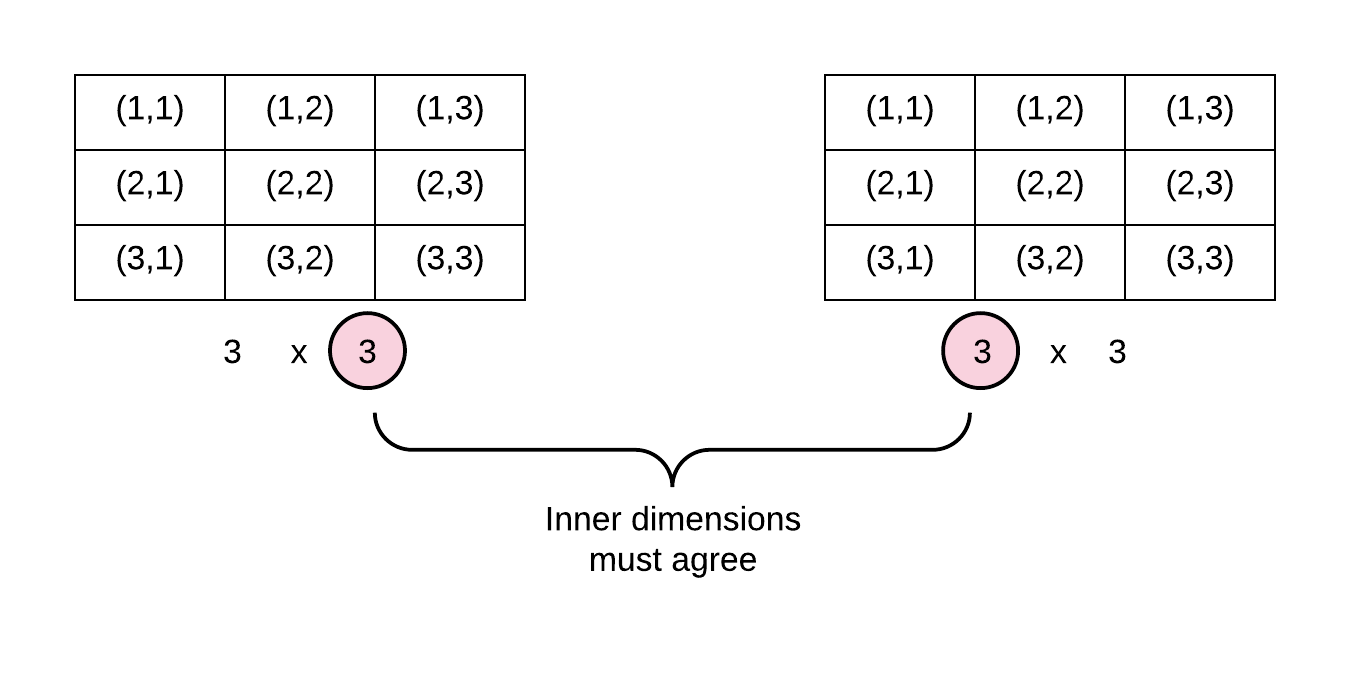Figure 1: Matrix multiplication
# multiply the two matrices A and B (dot product)
> A @ B    # or np.matmul(A,B)
'Output':
array([[2290, 2478, 2240],
[1784, 2072, 1792],
[3040, 3448, 2360]])


#### Element-wise operations

Element-wise matrix operations involve matrices operating on themselves in an element-wise fashion. The action can be an addition, subtraction, division or multiplication (which is commonly called the Hadamard product). The matrices must be of the same shape. Please note that while a matrix is of shape $n\; \times\; n\;$, a vector is of shape $n\; \times\; 1$. These concepts easily apply to vectors as well.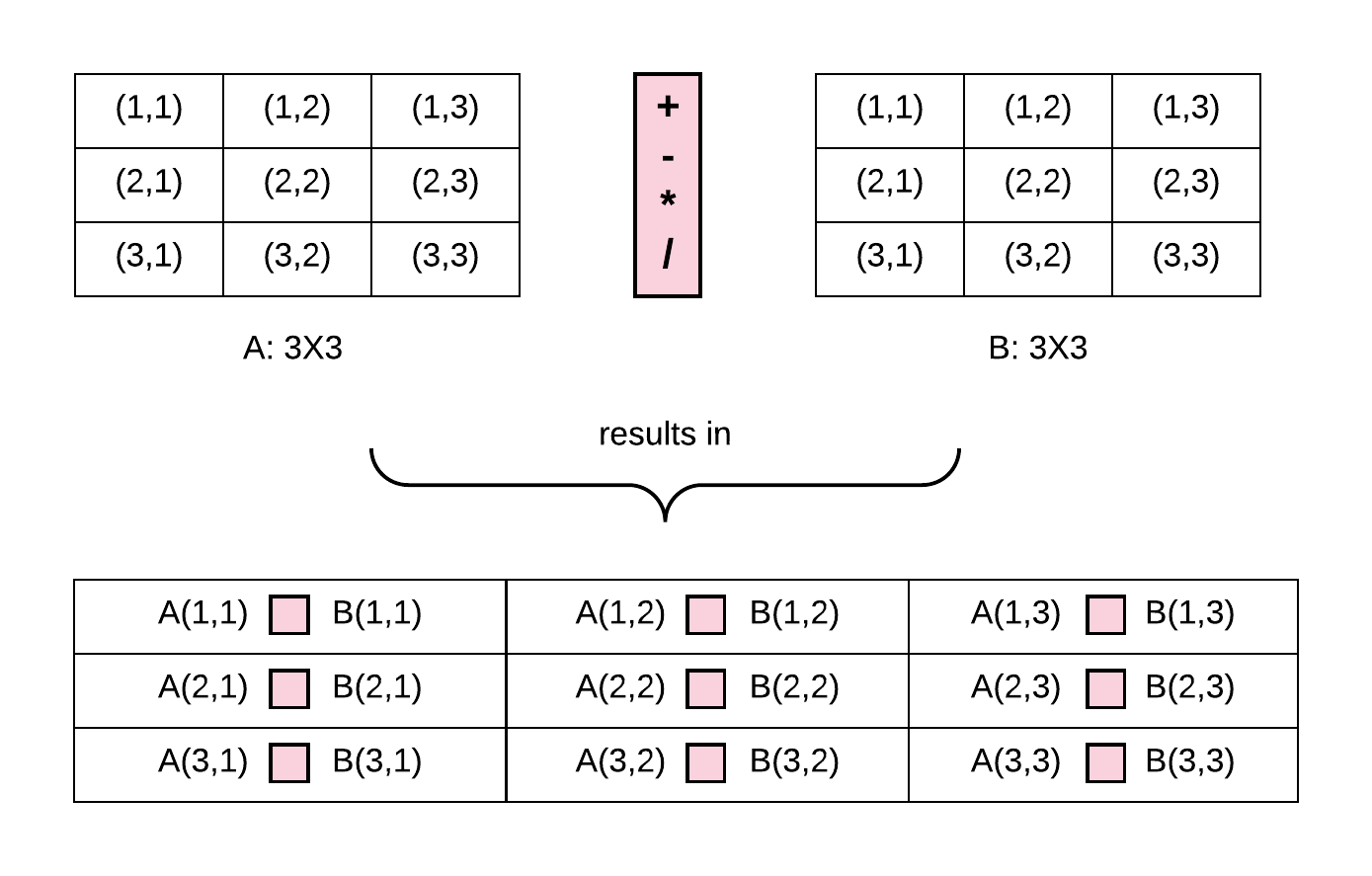Figure 2: Element-wise matrix operations

Let’s have some examples

# Hadamard multiplication of A and B
> A * B
'Output':
array([[ 570,  928,  528],
[ 160,  690, 1196],
[ 990,  658, 1056]])
> A + B
'Output':
array([[53, 61, 46],
[37, 53, 72],
[63, 61, 68]])
# subtract A from B
> B - A
'Output':
array([[ 23,   3,  -2],
[ 27,   7,  20],
[  3,  33, -20]])
# divide A with B
> A / B
'Output':
array([[ 0.39473684,  0.90625   ,  1.09090909],
[ 0.15625   ,  0.76666667,  0.56521739],
[ 0.90909091,  0.29787234,  1.83333333]])


#### Scalar Operation

A matrix can be acted upon by a scalar (i.e., a single numeric entity) in the same way element-wise fashion. This time the scalar operates upon each element of the matrix or vector.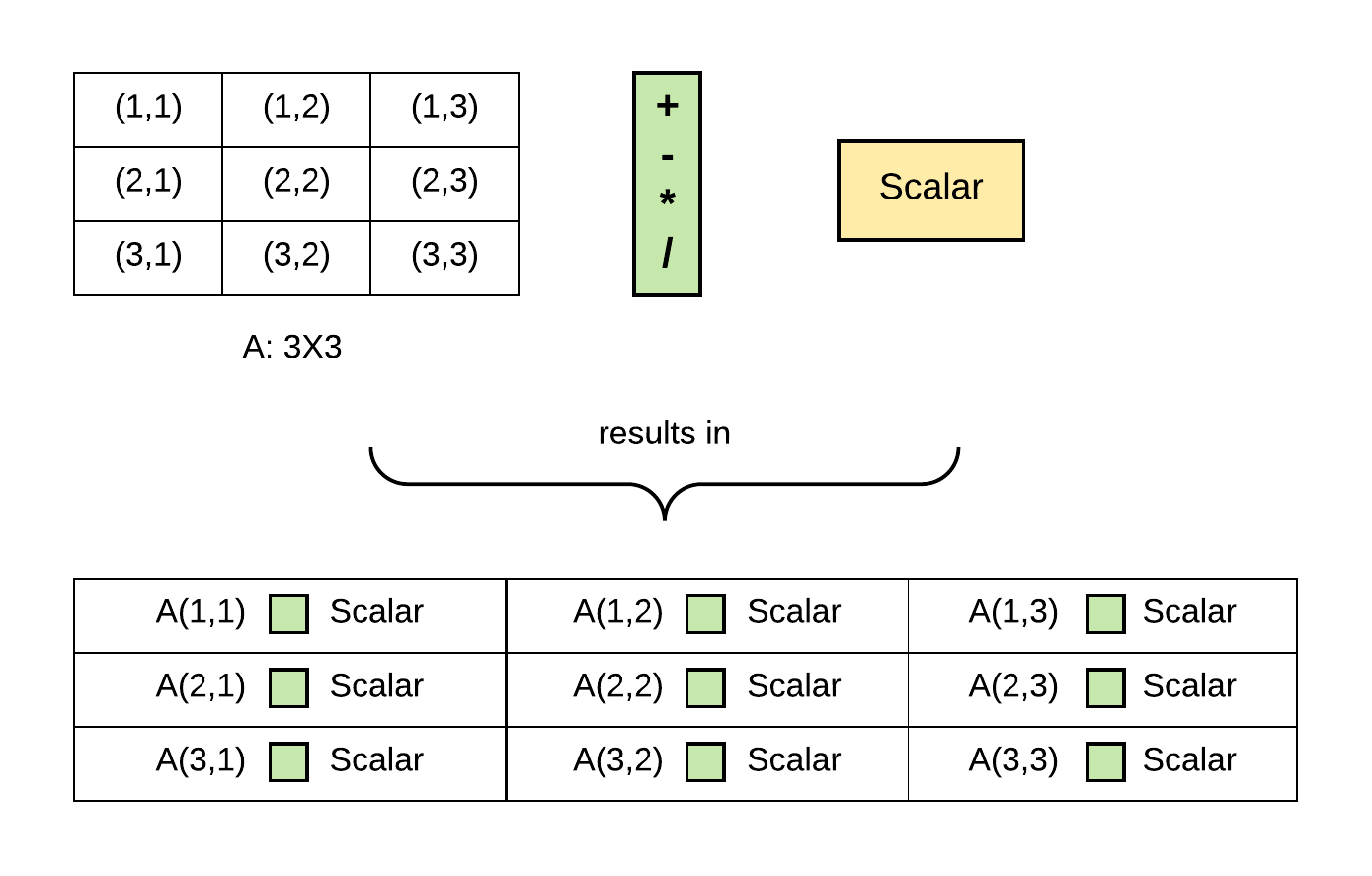Figure 3: Scalar operations

Let’s look at some examples

# Hadamard multiplication of A and a scalar, 0.5
> A * 0.5
'Output':
array([[  7.5,  14.5,  12. ],
[  2.5,  11.5,  13. ],
[ 15. ,   7. ,  22. ]])
# add A and a scalar, 0.5
> A + 0.5
'Output':
array([[ 15.5,  29.5,  24.5],
[  5.5,  23.5,  26.5],
[ 30.5,  14.5,  44.5]])
# subtract a scalar 0.5 from B
> B - 0.5
'Output':
array([[ 37.5,  31.5,  21.5],
[ 31.5,  29.5,  45.5],
[ 32.5,  46.5,  23.5]])
# divide A and a scalar, 0.5
> A / 0.5
'Output':
array([[ 30.,  58.,  48.],
[ 10.,  46.,  52.],
[ 60.,  28.,  88.]])


#### Matrix Transposition

Transposition is a vital matrix operation that reverses the rows and columns of a matrix by flipping the row and column indices. The transpose of a matrix is denoted as $A^T$. Observe that the diagonal elements remain unchanged.Figure 4: Matrix transpose

Let’s see an example

> A = np.array([[15, 29, 24],
[ 5, 23, 26],
[30, 14, 44]])
# transpose A
> A.T   # or A.transpose()
'Output':
array([[15,  5, 30],
[29, 23, 14],
[24, 26, 44]])


#### The Inverse of a Matrix

A $\;m \;\times\; m\;$ matrix $\;A\;$ (also called a square matrix) has an inverse if $A$ times another matrix $B$ results in the identity matrix $\;I\;$ also of shape $\;m \;\times\; m\;$. This matrix $B$ is called the inverse of $A$ and is denoted as $A^{-1}$. This relationship is formally written as:

However, not all matrices have an inverse. A matrix with an inverse is called a nonsingular or invertible matrix, while those without an inverse are known as singular or degenerate.

Note: A square matrix is a matrix that has the same number of rows and columns.

Let’s use NumPy to get the inverse of a matrix. Some linear algebra modules are found in a sub-module of NumPy called linalg.

> A = np.array([[15, 29, 24],
[ 5, 23, 26],
[30, 14, 44]])
# find the inverse of A
> np.linalg.inv(A)
'Output':
array([[ 0.05848375, -0.08483755,  0.01823105],
[ 0.05054152, -0.00541516, -0.02436823],
[-0.05595668,  0.05956679,  0.01805054]])


NumPy also implement the Moore-Penrose pseudo inverse, which gives an inverse derivation for degenerate matrices. Generally, it is preferred to use pinv to find the inverses of invertible matrices.

# using pinv()
> np.linalg.pinv(A)
'Output':
array([[ 0.05848375, -0.08483755,  0.01823105],
[ 0.05054152, -0.00541516, -0.02436823],
[-0.05595668,  0.05956679,  0.01805054]])



### Reshaping

A NumPy array can be restructured to take-on a different shape. Let’s convert a 1-D array to a $\;m \times n\;$ matrix

# make 20 elements evenly spaced between 0 and 5
> a = np.linspace(0,5,20)
> a
'Output':
array([ 0.        ,  0.26315789,  0.52631579,  0.78947368,  1.05263158,
1.31578947,  1.57894737,  1.84210526,  2.10526316,  2.36842105,
2.63157895,  2.89473684,  3.15789474,  3.42105263,  3.68421053,
3.94736842,  4.21052632,  4.47368421,  4.73684211,  5.        ])
# observe that a is a 1-D array
> a.shape
'Output': (20,)
# reshape into a 5 x 4 matrix
> A = a.reshape(5, 4)
> A
'Output':
array([[ 0.        ,  0.26315789,  0.52631579,  0.78947368],
[ 1.05263158,  1.31578947,  1.57894737,  1.84210526],
[ 2.10526316,  2.36842105,  2.63157895,  2.89473684],
[ 3.15789474,  3.42105263,  3.68421053,  3.94736842],
[ 4.21052632,  4.47368421,  4.73684211,  5.        ]])
# The vector a has been reshaped into a 5 by 4 matrix A
> A.shape
'Output': (5, 4)


#### Reshape vs. Resize Method

NumPy has the np.reshape and np.resize methods. The reshape method returns an ndarray with a modified shape without changing the original array, whereas the resize method changes the original array. Let’s see an example

# generate 9 elements evenly spaced between 0 and 5
> a = np.linspace(0,5,9)
> a
'Output':  array([ 0.   ,  0.625,  1.25 ,  1.875,  2.5  ,  3.125,  3.75 ,  4.375,  5.   ])
# the original shape
> a.shape
'Output':  (9,)
# call the resahpe method
> a.reshape(3,3)
'Output':
array([[ 0.   ,  0.625,  1.25 ],
[ 1.875,  2.5  ,  3.125],
[ 3.75 ,  4.375,  5.   ]])
# the original array maintained it's shape
> a.shape
'Output':  (9,)
# call the resize method - resize does not return an array
> a.resize(3,3)
# the resize method has changed the shape of the original array
> a.shape
'Output':  (3, 3)


#### Stacking Arrays

NumPy has methods for concatenating arrays - also called stacking. The methods hstack and vstack are used to stack several arrays along the horizontal and vertical axis respectively.

# create a 2x2 matrix of random integers in the range of 1 to 20
> A = np.random.randint(1, 50, size=[3,3])
> B = np.random.randint(1, 50, size=[3,3])
# print out the arrays
> A
'Output':
array([[19, 40, 31],
[ 5, 16, 38],
[22, 49,  9]])

> B
'Output':
array([[15, 22, 16],
[49, 26,  9],
[42, 13, 39]])


Let’s stack A and B horizontally using hstack. To use hstack, the arrays must have the same number of rows. Also, the arrays to be stacked are passed as a tuple to the hstack method.

# arrays are passed as tuple to hstack
> np.hstack((A,B))
'Output':
array([[19, 40, 31, 15, 22, 16],
[ 5, 16, 38, 49, 26,  9],
[22, 49,  9, 42, 13, 39]])


To stack A and B vertically using vstack the arrays must have the same number of columns. The arrays to be stacked are also passed as a tuple to the vstack method.

# arrays are passed as tuple to hstack
> np.vstack((A,B))
'Output':
array([[19, 40, 31],
[ 5, 16, 38],
[22, 49,  9],
[15, 22, 16],
[49, 26,  9],
[42, 13, 39]])


NumPy has an elegant mechanism for arithmetic operation on arrays with different dimensions or shapes. This is simply seen when a scalar is added to a vector (or 1-D array). The scalar value is conceptually broadcasted or stretched across the rows of the array and added element-wise.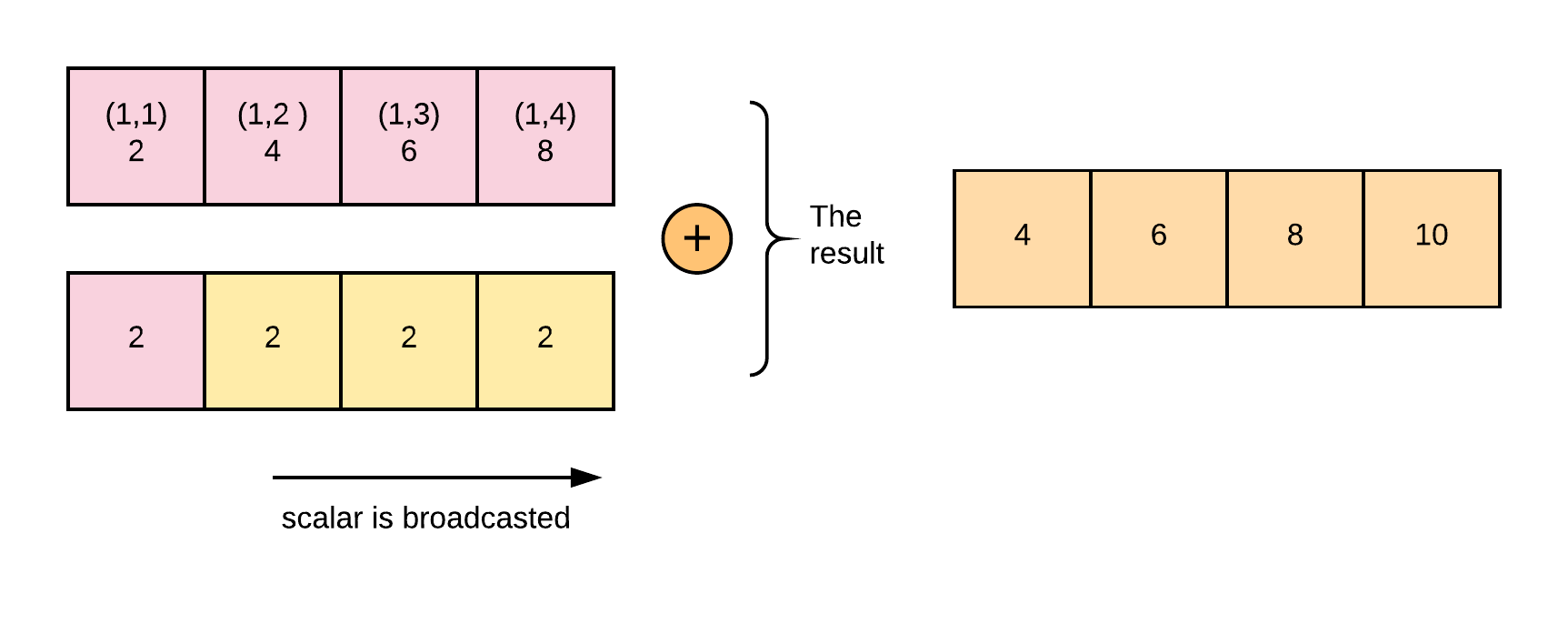Figure 4: Broadcasting example of adding a scalar to a vector (or 1-D array)

Matrices with different shapes can be broadcasted to perform arithmetic operations by stretching the dimension of the smaller array. Broadcasting is another vectorized operation for speeding up matrix processing. However, not all arrays with different shapes can be broadcasted. For broadcasting to occur, the trailing axes for the arrays must be the same size or 1.

In the example below, the matrix A and B have the same rows, but the columns of matrix B is 1. Hence an arithmetic operation can be performed on them by broadcasting and adding the cells element-wise.

A      (2d array):  4 x 3       + <perform addition>
B      (2d array):  4 x 1
Result (2d array):  4 x 3


See the figure below for more illustration.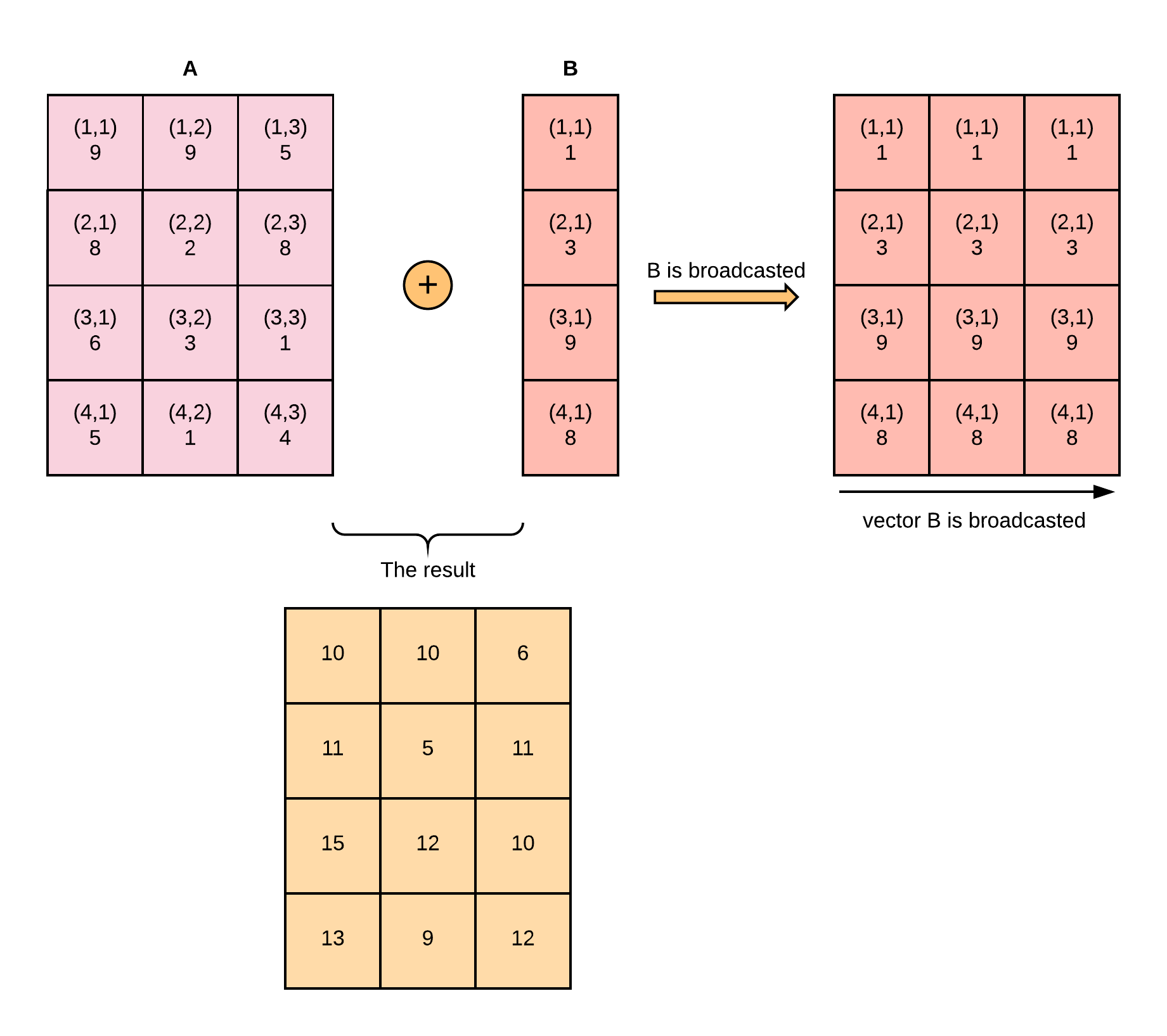Let’s see this in code:

# create a 4 X 3 matrix of random integers between 1 and 10
> A = np.random.randint(1, 10, [4, 3])
> A
'Output':
array([[9, 9, 5],
[8, 2, 8],
[6, 3, 1],
[5, 1, 4]])
# create a 4 X 1 matrix of random integers between 1 and 10
> B = np.random.randint(1, 10, [4, 1])
> B
'Output':
array([,
,
,
])
> A + B
'Output':
array([[10, 10,  6],
[11,  5, 11],
[15, 12, 10],
[13,  9, 12]])


The example below cannot be broadcasted and will result in a ValueError: operands could not be broadcast together with shapes (4,3) (4,2) because the matrix A and B have different columns and does not fit with the afore-mention rules of broadcasting that the trailing axes for the arrays must be the same size or 1.

A      (2d array):  4 x 3
B      (2d array):  4 x 2
The dimensions do not match - they must be either the same or 1


When we try to add the above example in Python, we get an error.

> A = np.random.randint(1, 10, [4, 3])
> B = np.random.randint(1, 10, [4, 2])
> A + B
'Output':
Traceback (most recent call last):

File "<ipython-input-145-624e41e41a31>", line 1, in <module>
A + B

ValueError: operands could not be broadcast together with shapes (4,3) (4,2)


Loading data is an important process in the data analysis/ machine learning pipeline. Data usually comes in .csv format. csv files can be loaded into Python by using the loadtxt method. The parameter skiprows skips the first row of the dataset - it is usually the header row of the data.
np.loadtxt(open("the_file_name.csv", "rb"), delimiter=",", skiprows=1)